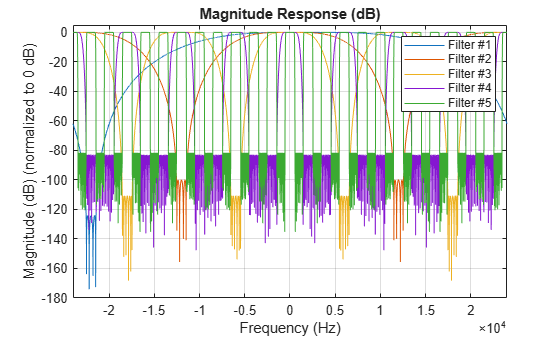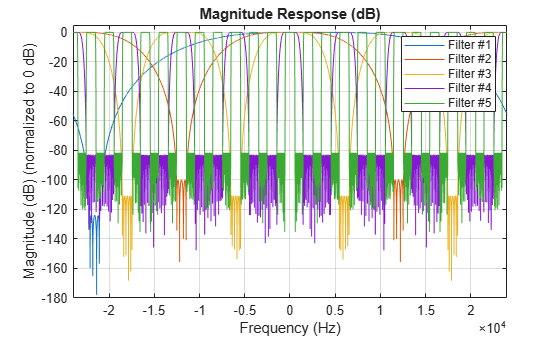# dsp.ComplexBandpassDecimator

Extract a frequency subband using a one-sided (complex) bandpass decimator

## Description

The `dsp.ComplexBandpassDecimator` System object™ extracts a specific sub-band of frequencies using a one-sided, multistage, complex bandpass decimator. The object determines the bandwidth of interest using the specified `CenterFrequency`, `DecimationFactor` and `Bandwidth` values.

To extract a frequency subband using a complex bandpass decimator:

1. Create the `dsp.ComplexBandpassDecimator` object and set its properties.

2. Call the object with arguments, as if it were a function.

This object supports C/C++ code generation and SIMD code generation under certain conditions. For more information, see Code Generation.

## Creation

### Syntax

``bpdecim = dsp.ComplexBandpassDecimator``
``bpdecim = dsp.ComplexBandpassDecimator(d)``
``bpdecim = dsp.ComplexBandpassDecimator(d,Fc)``
``bpdecim = dsp.ComplexBandpassDecimator(d,Fc,Fs)``
``bpdecim = dsp.ComplexBandpassDecimator(Name,Value)``

### Description

````bpdecim = dsp.ComplexBandpassDecimator` creates a System object that filters each channel of the input over time using a one-sided, multistage, complex bandpass decimation filter. The object determines the bandwidth of interest using the default center frequency, decimation factor, and bandwidth values.```

example

````bpdecim = dsp.ComplexBandpassDecimator(d)` creates a complex bandpass decimator object with the `DecimationFactor` property set to `d`.```

example

````bpdecim = dsp.ComplexBandpassDecimator(d,Fc)` creates a complex bandpass decimator object with the `DecimationFactor` property set to `d`, and the `CenterFrequency` property set to `Fc`.```

example

````bpdecim = dsp.ComplexBandpassDecimator(d,Fc,Fs)` creates a complex bandpass decimator object with the `DecimationFactor` property set to `d`, the `CenterFrequency` property set to `Fc`, and the `SampleRate` property set to `Fs`.Example: `dsp.ComplexBandpassDecimator(48e3/1e3,2e3,48e3);````
````bpdecim = dsp.ComplexBandpassDecimator(Name,Value)` creates a complex bandpass decimator object with each specified property set to the specified value. Enclose each property name in quotes. You can use this syntax with any previous input argument combinations.Example: `dsp.ComplexBandpassDecimator(48e3/1e3,2e3,48e3,'CenterFrequency',1e3);````

## Properties

expand all

Unless otherwise indicated, properties are nontunable, which means you cannot change their values after calling the object. Objects lock when you call them, and the `release` function unlocks them.

If a property is tunable, you can change its value at any time.

Center frequency of the desired band in Hz, specified as a real, finite numeric scalar in the range [-`SampleRate`/2, `SampleRate`/2].

Tunable: Yes

Data Types: `single` | `double`

Filter design parameters, specified as:

• `'Decimation factor'` –– The object specifies the decimation factor through the `Decimation Factor` property. The bandwidth of interest (BW) is computed using the following equation:

`$BW=Fs/D$`

where

• Fs –– Sample rate specified through `SampleRate` property.

• D –– Decimation factor.

• `'Bandwidth'` –– The object specifies the bandwidth through the `Bandwidth` property. The decimation factor (D) is computed using the following equation:

`$D=\text{floor}\left(\frac{Fs}{BW+TW}\right)$`

where

• Fs –– Sample rate specified through `SampleRate` property.

• BW –– Bandwidth of interest.

• TW –– Transition width specified through the `TransitionWidth` property.

• `'Decimation factor and bandwidth'` –– The decimation factor and the bandwidth of interest are specified through the `DecimationFactor` and `Bandwidth` properties.

Factor by which to reduce the bandwidth of the input signal, specified as a positive integer. The frame size (number of rows) of the input signal must be a multiple of the decimation factor.

#### Dependencies

This property applies when you set `Specification` to either `'Decimation factor'` or ```'Decimation factor and bandwidth'```.

Data Types: `single` | `double` | `int8` | `int16` | `int32` | `int64` | `uint8` | `uint16` | `uint32` | `uint64`

Stopband attenuation of the filter in dB, specified as finite positive scalar.

Data Types: `single` | `double` | `int8` | `int16` | `int32` | `int64` | `uint8` | `uint16` | `uint32` | `uint64`

Transition width of the filter in Hz, specified as a positive scalar.

Data Types: `single` | `double` | `int8` | `int16` | `int32` | `int64` | `uint8` | `uint16` | `uint32` | `uint64`

Width of the frequency band of interest, specified as a real positive scalar in Hz.

#### Dependencies

This property applies when you set `Specification` to either `'Bandwidth'` or ```'Decimation factor and bandwidth'```.

Data Types: `single` | `double`

Passband ripple of the filter, specified as a positive scalar in dB.

#### Dependencies

This property applies when you set `Specification` to either `'Bandwidth'` or ```'Decimation factor and bandwidth'```.

Data Types: `single` | `double` | `int8` | `int16` | `int32` | `int64` | `uint8` | `uint16` | `uint32` | `uint64`

Flag to minimize the number of complex filter coefficients, specified as:

• `true` –– The first stage of the multistage filter is bandpass (complex coefficients) centered at the specified center frequency. The first stage is followed by a mixing stage that heterodynes the signal to DC. The remaining filter stages, all with real coefficients, follow.

• `false` –– The input signal is first passed through the different stages of the multistage filter. All stages are bandpass (complex coefficients). The signal is then heterodyned to DC if `MixToBaseband` is `true`, and the frequency offset resulting from the decimation is nonzero.

Flag to mix the signal to baseband, specified as:

• `true` –– The object heterodynes the filtered, decimated signal to DC. This mixing stage runs at the output sample rate of the filter.

• `false` –– The object skips the mixing stage.

#### Dependencies

This property applies when you set `MinimizeComplexCoefficients` to `false`.

Sampling rate of the input signal in Hz, specified as a real positive scalar.

Data Types: `single` | `double`

## Usage

### Syntax

``y = bpdecim(x)``

### Description

````y = bpdecim(x)` filters the real or complex input signal, `x`, to produce the output, `y`. The output contains the subband of frequencies specified by the System object properties. The System object filters each channel of the input signal independently over time. The frame size (first dimension) of `x` must be a multiple of the decimation factor.```

### Input Arguments

expand all

Data input, specified as a vector or a matrix of size P-by-Q. The number of input rows P can be arbitrary and does not have to be a multiple of the decimation factor.

This object supports variable-size input signals, that is, the frame length (number of rows) of the signal can change even when the object is locked. However, the number of channels (columns) must remain constant.

Data Types: `single` | `double`
Complex Number Support: Yes

### Output Arguments

expand all

Output of the complex bandpass decimator, returned as a vector or a matrix. The output contains the subband of frequencies specified by the System object properties.

When the input is of size P-by-Q, and P is not a multiple of the decimation factor D, the output signal has an upper bound size of `ceil`(P/D)-by-Q, where D is the decimation factor. If P is a multiple of the decimation factor, then the output is of size (P/D)-by-Q. The number of channels (columns) does not change.

The data type of the output is same as the data type of the input. The output signal is always complex.

Data Types: `single` | `double`
Complex Number Support: Yes

## Object Functions

To use an object function, specify the System object as the first input argument. For example, to release system resources of a System object named `obj`, use this syntax:

`release(obj)`

expand all

 `cost` Implementation cost of the complex bandpass decimator `freqz` Frequency response of the multirate multistage filter `info` Information about filter System object `visualizeFilterStages` Visualize filter stages
 `step` Run System object algorithm `release` Release resources and allow changes to System object property values and input characteristics `reset` Reset internal states of System object

## Examples

collapse all

Compute the implementation cost of a complex bandpass decimator using the `cost` function.

Create a `dsp.ComplexBandpassDecimator` object. Set the `DecimationFactor` to 12, the `CenterFrequency` to 5000 Hz, and the `SampleRate` to 44,100 Hz.

`cbp = dsp.ComplexBandpassDecimator(12,5000,44100)`
```cbp = dsp.ComplexBandpassDecimator with properties: CenterFrequency: 5000 Specification: 'Decimation factor' DecimationFactor: 12 StopbandAttenuation: 80 TransitionWidth: 100 MinimizeComplexCoefficients: true SampleRate: 44100 ```

Compute the implementation cost of `cbp` using the `cost` function.

`c = cost(cbp)`
```c = struct with fields: NumCoefficients: 201 NumStates: 379 RealMultiplicationsPerInputSample: 44.3333 RealAdditionsPerInputSample: 43.8333 ```

Compute the complex frequency response of a complex bandpass decimator using the `freqz` function.

Create a `dsp.ComplexBandpassDecimator` object. Set the `DecimationFactor` to 12, the `CenterFrequency` to 5000 Hz, and the `SampleRate` to 44100 Hz. Compute and display the frequency response.

```cbp = dsp.ComplexBandpassDecimator(12,5000,44100); [h,f] = freqz(cbp); plot(f,20*log10(abs(h))) grid on xlabel('Frequency (Hz)') ylabel('h (dB)')```Filter an input signal through a complex bandpass decimator and visualize the filtered spectrum in a spectrum analyzer.

Initialization

Create a `dsp.ComplexBandpassDecimator` System object™ with center frequency set to 2000 Hz, bandwidth of interest set to 1000 Hz, and sample rate set to 48 kHz. The decimation factor is computed as the ratio of the sample rate to the bandwidth of interest. The input to the decimator is a sine wave with a frame length of 1200 samples with tones at 1625 Hz, 2000 Hz, and 2125 Hz. Create a `spectrumAnalyzer` scope to visualize the signal spectrum.

```Fs = 48e3; CF = 2000; BW = 1000; D = Fs/BW; FrameLength = 1200; bpdecim = dsp.ComplexBandpassDecimator(D,CF,Fs); sa = spectrumAnalyzer('SampleRate',Fs/D,... 'Method','welch',... 'YLimits',[-120 40],... 'FrequencyOffset',CF); tones = [1625 2000 2125]; sin = dsp.SineWave('SampleRate',Fs,'Frequency',tones,... 'SamplesPerFrame',FrameLength);```

Visualize Filter Stages

Using the `visualizeFilterStages` function, you can visualize the response of each individual filter stage using FVTool.

`visualizeFilterStages(bpdecim)`Display Filter `info`

The `info` function displays information about the bandpass decimator.

`fprintf('%s',info(bpdecim))`
```Overall Decimation Factor : 48 Bandwidth : 1000 Hz Number of Filters : 5 Real multiplications per Input Sample: 14.708333 Real additions per Input Sample : 13.833333 Number of Coefficients : 89 Filters: Filter 1: dsp.FIRDecimator - Decimation Factor : 2 Filter 2: dsp.FIRDecimator - Decimation Factor : 2 Filter 3: dsp.FIRDecimator - Decimation Factor : 2 Filter 4: dsp.FIRDecimator - Decimation Factor : 3 Filter 5: dsp.FIRDecimator - Decimation Factor : 2 ```

Stream In and Filter Signal

Construct a for-loop to run for 1000 iterations. In each iteration, stream in 1200 samples (one frame) of the noisy sine wave and apply the complex bandpass decimator on each frame of the input signal. Visualize the input and output spectrum in the spectrum analyzer, `sa`.

```for index = 1:1000 x = sum(sin(),2) + 1e-4*randn(FrameLength,1); z = bpdecim(x); sa(z); end```The bandpass decimator with center frequency at 2000 Hz and a bandwidth of 1000 Hz passes the three sine wave tones at 1625 Hz, 2000 Hz, and 2125 Hz.

Change the center frequency of the decimator to 2400 Hz and filter the signal.

```release(bpdecim); bpdecim.CenterFrequency = 2400```
```bpdecim = dsp.ComplexBandpassDecimator with properties: CenterFrequency: 2400 Specification: 'Decimation factor' DecimationFactor: 48 StopbandAttenuation: 80 TransitionWidth: 100 MinimizeComplexCoefficients: true SampleRate: 48000 ```

Configure the spectrum analyzer to show the bandwidth of interest, [-1900, 2900] Hz.

```release(sa) sa.FrequencyOffset = 2400;```Stream in the data and filter the signal.

```for index = 1:1000 x = sum(sin(),2) + 1e-4 * randn(FrameLength,1); z = bpdecim(x); sa(z); end```The tones at 2000 Hz and 2125 Hz are passed through the decimator, while the tone at 1625 Hz is filtered out.

## Algorithms

The complex bandpass decimator is designed by applying a complex frequency shift transformation on a lowpass prototype filter. The lowpass prototype in this case is a multirate, multistage finite impulse response (FIR) filter. The desired frequency shift applies only to the first stage. Subsequent stages scale the desired frequency shift by their respective cumulative decimation factors. For details, see Complex Bandpass Filter Design and Zoom FFT.

## Version History

Introduced in R2018a

expand all